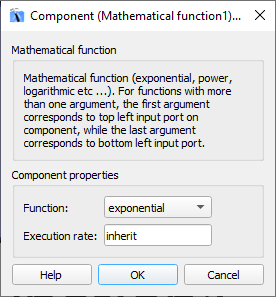# Mathematical Function

Description of the Mathematical Function component in Schematic Editor, which performs one of the selected mathematical functions on the input signal.

## Description

Performs one of the selected mathematical functions on the input signal.

The following mathematical operations are available:
• Exponential – calculates the exponential value of the input signal
• y(t)=ex(t)
• log10 – calculates the logarithm with base 10
• y(t)=log10 (x(t))
• ln – calculates the natural logarithm, that is the logarithm with base e
• y(t)=loge (x(t))=ln(x(t))
• square – calculates the value of the input to the power of 2
• y(t)=x(t)2
• power – calculates the value of the input to the power of v, where v is defined by the second input signal
• y(t)=x(t)u(t)
• sqrt – calculates the square root of the input signal
• y(t)=sqrt(x(t))
• reciprocal – calculates the reciprocal value of the input signal
• y(t)=1/x(t)

Input signals can be scalars or vectors. If signals are vectors, operations are done element-wise

## Ports

• in (in)
• The input of the component.
• Supported types: uint, int and real.
• Vector support: yes.
• v (in)
• The input of the component is only available when power operation is being performed. In this case, this input is the exponent.
• Supported types: uint, int and real.
• Vector support: yes.
• Dynamically created when Function property is selected as ‘power’
• out (out)
• Result of the operation performed by the mathematical function.
• Supported types: real.
• Vector support: yes.
• The vector length is inherited from input signals.

## Properties• Function
• Select the operation that will be performed by the mathematical function component. Available operations are exponential, base 10 logarithm, natural logarithm, exponentiation, power, square root, and reciprocal function.
• Execution rate
• Type in the desired signal processing execution rate. This value must be compatible with other signal processing components of the same circuit: the value must be a multiple of the fastest execution rate in the circuit. There can be up to four different execution rates, but they must all be multiple of the basic simulation timestep. To specify the execution rate, you can use either decimal (e.g. 0.001) or exponential values (e.g. 1e-3) in seconds. Alternatively, you can type in ‘inherit’ in which case the component will be assigned execution rate based on the execution rate of the components it is receiving input from.